## Example Questions

1 2 304 305 306 307 308 309 310 312 Next →

### Example Question #31 : How To Solve For A Variable As Part Of A Fraction

Solve for.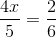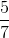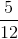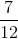Explanation:

First, cross-multiply.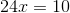Now, solve for.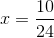Simplify.### Example Question #32 : How To Solve For A Variable As Part Of A Fraction

Solve for.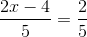Explanation:

First, cross-multiply.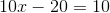Now, solve for.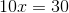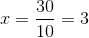### Example Question #81 : Algebraic Fractions

Solve for.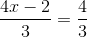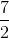Explanation:

First, cross-multiply.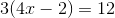Simplify.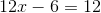Solve for.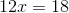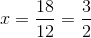### Example Question #82 : Algebraic Fractions

Solve for.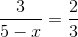Explanation:

First, cross-multiply.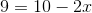Now, solve for.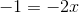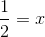### Example Question #91 : Algebraic Fractions

Solve for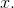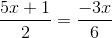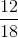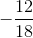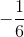Explanation:

To solve these types of problems, you need to cross multiply the fractions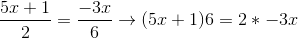.

Then, all we need to do is distribute the, combine like terms, and solve the algebraic equation.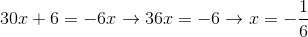.

### Example Question #92 : Algebraic Fractions

Solve for: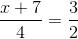Explanation:

Cross-multiply: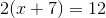Distribute: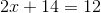Solve for x: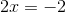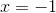### Example Question #93 : Algebraic Fractions

Solve for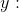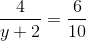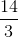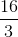Explanation:

Solve this question by cross-multiplying: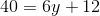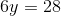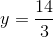### Example Question #3241 : Sat Mathematics

If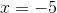, what is the value of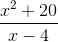?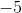Explanation:

To solve this question, substitute -5 in for x in the numerator and denominator. Remember that the square of a negative number is positive.

45 / -9 = -5

1 2 304 305 306 307 308 309 310 312 Next →

### All ACT Math Resources